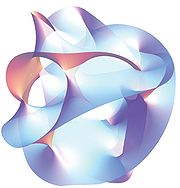A graph in mathematics is a collection of nodes connected with edges.  The degree of a node is the number of nodes directly connected to it. If one sees mathematics as a sort of graph where each node is a sub-domain, then the degree of probability theory is very high. Probability theory is directly connected to most areas of mathematics and appears as a sort of hub. Random matrix theory (probability theory mixed with linear algebra) has an impressive degree. Therefore, if you are young and open minded, it can be a good idea to start your mathematical life doing probability. This will allow you to travel easily across mathematics and beyond.

In the scientific life of a curious scientist, the starting point is less important than the personal evolution dynamics, but has some impact on it. If your curiosity is limited, you will maybe stay in a  neighborhood of some node, and you should select it carefully according to your tastes.  There is also the common possibility to  jump from one node to another two or three times in a scientific life. Mathematics need all sorts of mathematicians, not only probabilists. In practice, the teaching quality has an enormous impact on the starting point of young scientists. Seduction and randomness.By forgetting discrete aspects, one can also view mathematics as a high dimensional singular Riemannian manifold or length space where each point is a mathematical statement. A proof is a path, and a geodesic is a minimal length proof. There is a cut-locus phenomenon which corresponds to the existence of multiple minimal length proofs. Mathematicians are explorers on this curved word, climbing mountains and following valleys. Some of them have visions. The topology of this manifold involves gaps  reinforcing obstructions.Mathematics are everywhere in the daily life, but there is no “Maths inside” logo. Instead of opposing  pure and applied mathematics, one may adopt a global transverse vision based on concepts and implementations. One should drive its mathematical activity by problems rather than by techniques. One should learn and forge techniques to solve problems. One should take the time to write down expository texts and syntheses on mature subjects.

Last Updated on

This site uses Akismet to reduce spam. Learn how your comment data is processed.

Syntax · Style · .